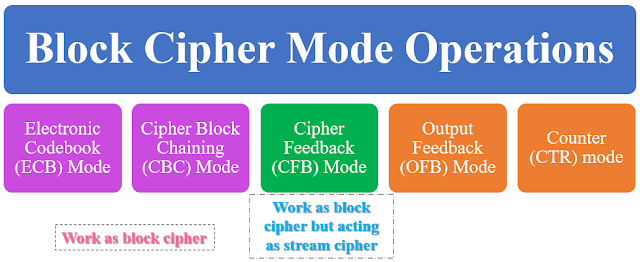## Friday, October 15, 2021

### Block Cipher Modes | Modes of Operation in Block Cipher | Application of Block Cipher Modes

Introduction

Cryptographic algorithm works on main two techniques: block and stream ciphers.

In a stream cipher, the plaintext is encrypted one bit at a time. In a block cipher, the plaintext is broken into blocks of a fixed length and the bits in each block are encrypted together. One of the main issues with block ciphers is that they only allow you to encrypt messages the fixed size as their block length.

If plaintext, which has a block size 64 bits easily encrypt. But encrypt a 65-bit message, you need a way to define how the second block should be encrypted.

The solution to this is called block cipher modes of operation. Need of block cipher mode is basic building block for providing data security. In block cipher rather than encrypting one bit at a time, block of bits is encrypted at a time.

There are 5 modes of operation for block cipher that may be used in a wide variety of applications like symmetric key cryptographic algorithm. These modes define how data encrypted and decrypted.Figure: Different Modes of Block Cipher

Application/Real time usage of block cipher modes operations